Courses

# Graph Theory - 1

## 10 Questions MCQ Test Networking Theory | Graph Theory - 1

Description
This mock test of Graph Theory - 1 for Electrical Engineering (EE) helps you for every Electrical Engineering (EE) entrance exam. This contains 10 Multiple Choice Questions for Electrical Engineering (EE) Graph Theory - 1 (mcq) to study with solutions a complete question bank. The solved questions answers in this Graph Theory - 1 quiz give you a good mix of easy questions and tough questions. Electrical Engineering (EE) students definitely take this Graph Theory - 1 exercise for a better result in the exam. You can find other Graph Theory - 1 extra questions, long questions & short questions for Electrical Engineering (EE) on EduRev as well by searching above.
QUESTION: 1

### Consider the following circuits :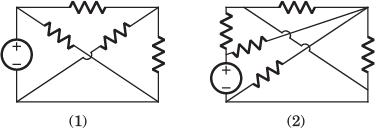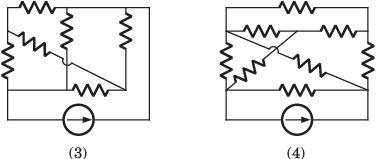The planner circuits are

Solution:

The circuit 1 and 2 are redrawn as below. 3 and 4 can not be redrawn on a plane without crossing other branch.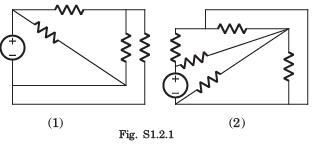QUESTION: 2

### Consider the following graphs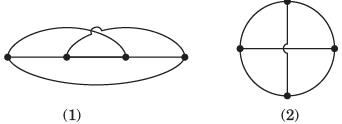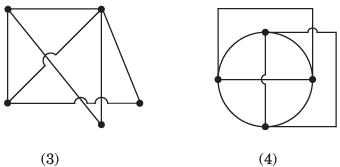Non-planner graphs are

Solution:

Other three circuits can be drawn on plane without crossing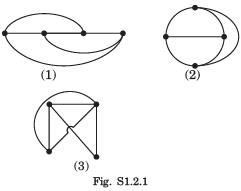QUESTION: 3

### A graph of an electrical network has 4 nodes and 7 branches. The number of links l, with respect to the chosen tree, would be

Solution:

l = b - ( n - 1) = 4.

QUESTION: 4

For the graph shown in fig. P.1.1.4 correct set is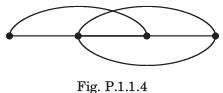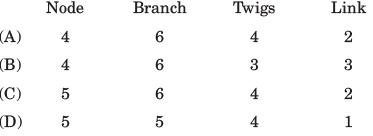Solution:

There are 4 node and 6 branches.
t = n - 1 = 3, l = b - n + 1 = 3

QUESTION: 5

A tree of the graph shown in fig. P.1.2.5 is​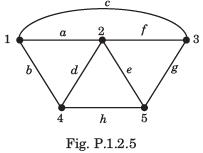Solution:

From fig. it can be seen that afhg is a tree of given graph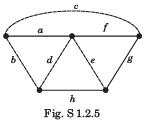QUESTION: 6

Branch current and loop current relation are expressed in matrix form as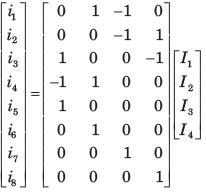where irepresent branch current and Ik loop current. The number of independent node equation are

Solution:

Number of branch = 8
Number of twigs = 8 - 4 = 4
Number of twigs =number of independent node equation.

QUESTION: 7

If the number of branch in a network is b, the number of nodes is n and the number of dependent loop is l, then the number of independent node equations will be

Solution:

The number of independent node equation are n - 1.

QUESTION: 8

Branch current and loop current relation are expressed in matrix form as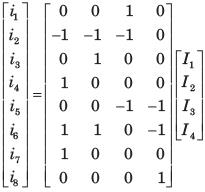where ij represent branch current and Ik loop current.
Q. The rank of incidence matrix is

Solution:

Number of branch b=8
Number of link l = 4
Number of twigs t = b - l = 4
rank of matrix = n - 1 = t = 4

QUESTION: 9

Branch current and loop current relation are expressed in matrix form aswhere ij represent branch current and Ik loop current.
Q. The directed graph will be

Solution:

We know the branch current and loop current are related as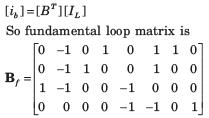f-loop 1 include branch (2, 4, 6, 7) and direction of branch–2 is opposite to other (B only).

QUESTION: 10

A network has 8 nodes and 5 independent loops.The number of branches in the network is

Solution: